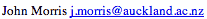Data Structures and Algorithms 10.2 Dijkstra's Algorithm

Djikstra's algorithm (named after its discover, E.W. Dijkstra) solves the problem of finding the shortest path from a point in a graph (the source) to a destination. It turns out that one can find the shortest paths from a given source to all points in a graph in the same time, hence this problem is sometimes called the single-source shortest paths problem.

The somewhat unexpected result that all the paths can be found as easily as one further demonstrates the value of reading the literature on algorithms!

This problem is related to the spanning tree one. The graph representing all the paths from one vertex to all the others must be a spanning tree - it must include all vertices. There will also be no cycles as a cycle would define more than one path from the selected vertex to at least one other vertex. For a graph,

 G = (V,E) where V is a set of vertices and E is a set of edges.
Dijkstra's algorithm keeps two sets of vertices:
 S the set of vertices whose shortest paths from the source have already been determined and V-S the remaining vertices.
The other data structures needed are:
 d array of best estimates of shortest path to each vertex pi an array of predecessors for each vertex

The basic mode of operation is:

1. Initialise d and pi,
2. Set S to empty,
3. While there are still vertices in V-S,
1. Sort the vertices in V-S according to the current best estimate of their distance from the source,
2. Add u, the closest vertex in V-S, to S,
3. Relax all the vertices still in V-S connected to u

#### Relaxation

The relaxation process updates the costs of all the vertices, v, connected to a vertex, u, if we could improve the best estimate of the shortest path to v by including (u,v) in the path to v.

The relaxation procedure proceeds as follows:

```initialise_single_source( Graph g, Node s )
for each vertex v in Vertices( g )
g.d[v] := infinity
g.pi[v] := nil
g.d[s] := 0;
```
This sets up the graph so that each node has no predecessor (pi[v] = nil) and the estimates of the cost (distance) of each node from the source (d[v]) are infinite, except for the source node itself (d[s] = 0).

 Note that we have also introduced a further way to store a graph (or part of a graph - as this structure can only store a spanning tree), the predecessor sub-graph - the list of predecessors of each node, pi[j], 1 <= j <= |V| The edges in the predecessor sub-graph are (pi[v],v).

The relaxation procedure checks whether the current best estimate of the shortest distance to v (d[v]) can be improved by going through u (i.e. by making u the predecessor of v):

```relax( Node u, Node v, double w[][] )
if d[v] > d[u] + w[u,v] then
d[v] := d[u] + w[u,v]
pi[v] := u
```
The algorithm itself is now:
```shortest_paths( Graph g, Node s )
initialise_single_source( g, s )
S := { 0 }        /* Make S empty */
Q := Vertices( g ) /* Put the vertices in a PQ */
while not Empty(Q)
u := ExtractCheapest( Q );
for each vertex v in Adjacent( u )
relax( u, v, w )
```

As usual, proof of a greedy algorithm is the trickiest part.

### Animation

In this animation, a number of cases have been selected to show all aspects of the operation of Dijkstra's algorithm. Start by selecting the data set (or you can just work through the first one - which appears by default). Then select either step or run to execute the algorithm. Note that it starts by assigning a weight of infinity to all nodes, and then selecting a source and assigning a weight of zero to it. As nodes are added to the set for which shortest paths are known, their colour is changed to red. When a node is selected, the weights of its neighbours are relaxed .. nodes turn green and flash as they are being relaxed. Once all nodes are relaxed, their predecessors are updated, arcs are turned green when this happens. The cycle of selection, weight relaxation and predecessor update repeats itself until all the shortest path to all nodes has been found.

Dijkstra's Algorithm Animation
This animation was written by Mervyn Ng and Woi Ang.©, 1998